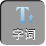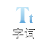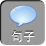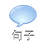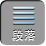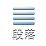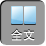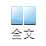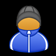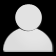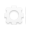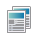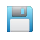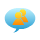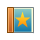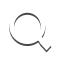-AA+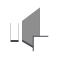1化简：( x+ 3 y-( y -2 x)

2化简： 4x+ 2 x 2 -5-2( 2 x-x 2+ 1).

3化简： 3( a 2+ 3) -2a 2 - a-( a 2+ 3)+ a 2 - a.

= 2( a 2+ 3) -a 2 - a）= 2 a 2+ 6 - a 2+ a= a 2+ a+ 6.

4化简： -6[（ x 2 -1-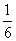( 2 x 2+ x) -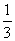( x -5)].

= -6 x 2+ 6-2 x 2 - x+ 2 x -10= -8 x 2+ x -4.

5化简: m 2 n -{ 3 mn 2 -[ 4 mn 2 -( 2 m 2 n-mn 2) -3 m 2 n]}.